# NCERT solutions for Mathematics Exemplar Class 9 chapter 7 - Triangles [Latest edition]

#### Chapters## Chapter 7: Triangles

Exercise 7.1Exercise 7.2Exercise 7.3Exercise 7.4
Exercise 7.1 [Page 64]

### NCERT solutions for Mathematics Exemplar Class 9 Chapter 7 Triangles Exercise 7.1 [Page 64]

#### Choose the correct alternative:

Exercise 7.1 | Q 1 | Page 64

Which of the following is not a criterion for congruence of triangles?

• SAS

• ASA

• SSA

• SSS

Exercise 7.1 | Q 2 | Page 64

If AB = QR, BC = PR and CA = PQ, then ______.

• ∆ABC ≅ ∆PQR

• ∆CBA ≅ ∆PRQ

• ∆BAC ≅ ∆RPQ

• ∆PQR ≅ ∆BCA

Exercise 7.1 | Q 3 | Page 64

In ∆ABC, AB = AC and ∠B = 50°. Then ∠C is equal to ______.

• 40°

• 50°

• 80°

• 130°

Exercise 7.1 | Q 4 | Page 64

In ∆ABC, BC = AB and ∠B = 80°. Then ∠A is equal to ______.

• 80°

• 40°

• 50°

• 100°

Exercise 7.1 | Q 5 | Page 64

In ∆PQR, ∠R = ∠P and QR = 4 cm and PR = 5 cm. Then the length of PQ is ______.

• 4 cm

• 5 cm

• 2 cm

• 2.5 cm

Exercise 7.1 | Q 6 | Page 64

D is a point on the side BC of a ∆ABC such that AD bisects ∠BAC. Then ______.

• BD = CD

• BA > BD

• BD > BA

• CD > CA

Exercise 7.1 | Q 7 | Page 64

It is given that ∆ABC ≅ ∆FDE and AB = 5 cm, ∠B = 40° and ∠A = 80°. Then which of the following is true?

• DF = 5 cm, ∠F = 60°

• DF = 5 cm, ∠E = 60°

• DE = 5 cm, ∠E = 60°

• DE = 5 cm, ∠D = 40°

Exercise 7.1 | Q 8 | Page 64

Two sides of a triangle are of lengths 5 cm and 1.5 cm. The length of the third side of the triangle cannot be ______.

• 3.6 cm

• 4.1 cm

• 3.8 cm

• 3.4 cm

Exercise 7.1 | Q 9 | Page 64

In ∆PQR, if ∠R > ∠Q, then ______.

• QR > PR

• PQ > PR

• PQ < PR

• QR < PR

Exercise 7.1 | Q 10 | Page 64

In triangles ABC and PQR, AB = AC, ∠C = ∠P and ∠B = ∠Q. The two triangles are ______.

• Isosceles but not congruent

• Isosceles and congruent

• Congruent but not isosceles

• Neither congruent nor isosceles

Exercise 7.1 | Q 11 | Page 64

In triangles ABC and DEF, AB = FD and ∠A = ∠D. The two triangles will be congruent by SAS axiom if ______.

• BC = EF

• AC = DE

• AC = EF

• BC = DE

Exercise 7.2 [Pages 65 - 66]

### NCERT solutions for Mathematics Exemplar Class 9 Chapter 7 Triangles Exercise 7.2 [Pages 65 - 66]

Exercise 7.2 | Q 1 | Page 65

In triangles ABC and PQR, ∠A = ∠Q and ∠B = ∠R. Which side of ∆PQR should be equal to side AB of ∆ABC so that the two triangles are congruent? Give reason for your answer.

Exercise 7.2 | Q 2 | Page 65

In triangles ABC and PQR, ∠A = ∠Q and ∠B = ∠R. Which side of ∆PQR should be equal to side BC of ∆ABC so that the two triangles are congruent? Give reason for your answer.

Exercise 7.2 | Q 3 | Page 65

“If two sides and an angle of one triangle are equal to two sides and an angle of another triangle, then the two triangles must be congruent.” Is the statement true? Why?

Exercise 7.2 | Q 4 | Page 65

“If two angles and a side of one triangle are equal to two angles and a side of another triangle, then the two triangles must be congruent.” Is the statement true? Why?

Exercise 7.2 | Q 5 | Page 65

Is it possible to construct a triangle with lengths of its sides as 4 cm, 3 cm and 7 cm? Give reason for your answer.

Exercise 7.2 | Q 6 | Page 65

It is given that ∆ABC ≅ ∆RPQ. Is it true to say that BC = QR? Why?

Exercise 7.2 | Q 7 | Page 65

If ∆PQR ≅ ∆EDF, then is it true to say that PR = EF? Give reason for your answer

Exercise 7.2 | Q 8 | Page 65

In ∆PQR, ∠P = 70° and ∠R = 30°. Which side of this triangle is the longest? Give reason for your answer

Exercise 7.2 | Q 9 | Page 65

AD is a median of the triangle ABC. Is it true that AB + BC + CA > 2 AD? Give reason for your answer.

Exercise 7.2 | Q 10 | Page 65

M is a point on side BC of a triangle ABC such that AM is the bisector of ∠BAC. Is it true to say that perimeter of the triangle is greater than 2 AM? Give reason for your answer

Exercise 7.2 | Q 11 | Page 66

Is it possible to construct a triangle with lengths of its sides as 9 cm, 7 cm and 17 cm? Give reason for your answer.

Exercise 7.2 | Q 12 | Page 66

Is it possible to construct a triangle with lengths of its sides as 8 cm, 7 cm and 4 cm? Give reason for your answer.

Exercise 7.3 [Pages 67 - 68]

### NCERT solutions for Mathematics Exemplar Class 9 Chapter 7 Triangles Exercise 7.3 [Pages 67 - 68]

Exercise 7.3 | Q 1 | Page 67

ABC is an isosceles triangle with AB = AC and BD and CE are its two medians. Show that BD = CE.

Exercise 7.3 | Q 2 | Page 67

In figure, D and E are points on side BC of a ∆ABC such that BD = CE and AD = AE. Show that ∆ABD ≅ ∆ACE.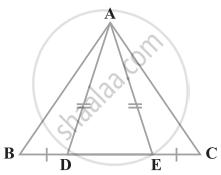Exercise 7.3 | Q 3 | Page 67

In the figure, ΔCDE is an equilateral triangle formed on a side CD of a square ABCD. Show that ∆ADE ≅ ∆BCE.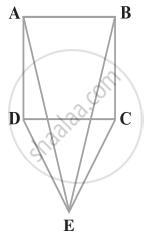Exercise 7.3 | Q 4 | Page 67

In the figure, BA ⊥ AC, DE ⊥ DF such that BA = DE and BF = EC. Show that ∆ABC ≅ ∆DEF.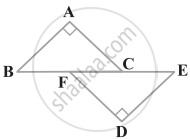Exercise 7.3 | Q 5 | Page 67

Q is a point on the side SR of a ∆PSR such that PQ = PR. Prove that PS > PQ.

Exercise 7.3 | Q 6 | Page 67

S is any point on side QR of a ∆PQR. Show that: PQ + QR + RP > 2PS.

Exercise 7.3 | Q 7 | Page 67

D is any point on side AC of a ∆ABC with AB = AC. Show that CD < BD.

Exercise 7.3 | Q 8 | Page 67

In given figure, l || m and M is the mid-point of a line segment AB. Show that M is also the mid-point of any line segment CD, having its endpoints on l and m, respectively.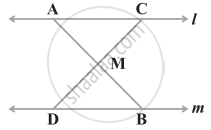Exercise 7.3 | Q 9 | Page 67

Bisectors of the angles B and C of an isosceles triangle with AB = AC intersect each other at O. BO is produced to a point M. Prove that ∠MOC = ∠ABC.

Exercise 7.3 | Q 10 | Page 68

Bisectors of the angles B and C of an isosceles triangle ABC with AB = AC intersect each other at O. Show that external angle adjacent to ∠ABC is equal to ∠BOC

Exercise 7.3 | Q 11 | Page 68

In the figure, AD is the bisector of ∠BAC. Prove that AB > BD.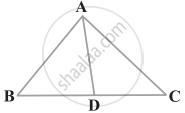Exercise 7.4 [Pages 69 - 71]

### NCERT solutions for Mathematics Exemplar Class 9 Chapter 7 Triangles Exercise 7.4 [Pages 69 - 71]

Exercise 7.4 | Q 1 | Page 69

Find all the angles of an equilateral triangle.

Exercise 7.4 | Q 2 | Page 69

The image of an object placed at a point A before a plane mirror LM is seen at the point B by an observer at D as shown in figure. Prove that the image is as far behind the mirror as the object is in front of the mirror.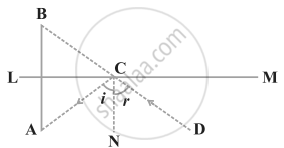Exercise 7.4 | Q 3 | Page 70

ABC is an isosceles triangle with AB = AC and D is a point on BC such that AD ⊥ BC (figure). To prove that ∠BAD = ∠CAD, a student proceeded as follows: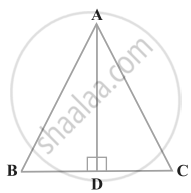In ∆ABD and ∆ACD,
AB = AC (Given)
∠B = ∠C (Because AB = AC)
Therefore, ∆ABD ≅ ∆ACD (AAS)
What is the defect in the above arguments?

Exercise 7.4 | Q 4 | Page 70

P is a point on the bisector of ∠ABC. If the line through P, parallel to BA meet BC at Q, prove that BPQ is an isosceles triangle.

Exercise 7.4 | Q 5 | Page 70

ABCD is a quadrilateral in which AB = BC and AD = CD. Show that BD bisects both the angles ABC and ADC.

Exercise 7.4 | Q 6 | Page 70

ABC is a right triangle with AB = AC. Bisector of ∠A meets BC at D. Prove that BC = 2AD.

Exercise 7.4 | Q 7 | Page 70

O is a point in the interior of a square ABCD such that OAB is an equilateral triangle. Show that ∆OCD is an isosceles triangle.

Exercise 7.4 | Q 8 | Page 70

ABC and DBC are two triangles on the same base BC such that A and D lie on the opposite sides of BC, AB = AC and DB = DC. Show that AD is the perpendicular bisector of BC.

Exercise 7.4 | Q 9 | Page 70

ABC is an isosceles triangle in which AC = BC. AD and BE are respectively two altitudes to sides BC and AC. Prove that AE = BD.

Exercise 7.4 | Q 10 | Page 70

Prove that sum of any two sides of a triangle is greater than twice the median with respect to the third side.

Exercise 7.4 | Q 11 | Page 70

Show that in a quadrilateral ABCD, AB + BC + CD + DA < 2(BD + AC)

Exercise 7.4 | Q 12 | Page 70

Show that in a quadrilateral ABCD, AB + BC + CD + DA > AC + BD

Exercise 7.4 | Q 13 | Page 70

In a triangle ABC, D is the mid-point of side AC such that BD = 1/2 AC. Show that ∠ABC is a right angle.

Exercise 7.4 | Q 14 | Page 70

In a right triangle, prove that the line-segment joining the mid-point of the hypotenuse to the opposite vertex is half the hypotenuse.

Exercise 7.4 | Q 15 | Page 71

Two lines l and m intersect at the point O and P is a point on a line n passing through the point O such that P is equidistant from l and m. Prove that n is the bisector of the angle formed by l and m.

Exercise 7.4 | Q 16 | Page 71

Line segment joining the mid-points M and N of parallel sides AB and DC, respectively of a trapezium ABCD is perpendicular to both the sides AB and DC. Prove that AD = BC.

Exercise 7.4 | Q 17 | Page 71

ABCD is a quadrilateral such that diagonal AC bisects the angles A and C. Prove that AB = AD and CB = CD.

Exercise 7.4 | Q 18 | Page 71

ABC is a right triangle such that AB = AC and bisector of angle C intersects the side AB at D. Prove that AC + AD = BC.

Exercise 7.4 | Q 19 | Page 71

AB and CD are the smallest and largest sides of a quadrilateral ABCD. Out of ∠B and ∠D decide which is greater

Exercise 7.4 | Q 20 | Page 71

Prove that in a triangle, other than an equilateral triangle, angle opposite the longest side is greater than 2/3 of a right angle.

Exercise 7.4 | Q 21 | Page 71

ABCD is quadrilateral such that AB = AD and CB = CD. Prove that AC is the perpendicular bisector of BD.

## Chapter 7: Triangles

Exercise 7.1Exercise 7.2Exercise 7.3Exercise 7.4## NCERT solutions for Mathematics Exemplar Class 9 chapter 7 - Triangles

NCERT solutions for Mathematics Exemplar Class 9 chapter 7 (Triangles) include all questions with solution and detail explanation. This will clear students doubts about any question and improve application skills while preparing for board exams. The detailed, step-by-step solutions will help you understand the concepts better and clear your confusions, if any. Shaalaa.com has the CBSE Mathematics Exemplar Class 9 solutions in a manner that help students grasp basic concepts better and faster.

Further, we at Shaalaa.com provide such solutions so that students can prepare for written exams. NCERT textbook solutions can be a core help for self-study and acts as a perfect self-help guidance for students.

Concepts covered in Mathematics Exemplar Class 9 chapter 7 Triangles are Concept of Triangles - Sides, Angles, Vertices, Interior and Exterior of Triangle, Properties of a Triangle, Some More Criteria for Congruence of Triangles, Inequalities in a Triangle, Criteria for Congruence of Triangles, Congruence of Triangles.

Using NCERT Class 9 solutions Triangles exercise by students are an easy way to prepare for the exams, as they involve solutions arranged chapter-wise also page wise. The questions involved in NCERT Solutions are important questions that can be asked in the final exam. Maximum students of CBSE Class 9 prefer NCERT Textbook Solutions to score more in exam.

Get the free view of chapter 7 Triangles Class 9 extra questions for Mathematics Exemplar Class 9 and can use Shaalaa.com to keep it handy for your exam preparation